`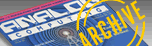A.N.A.L.O.G. ISSUE 72 / MAY 1989 / PAGE 27`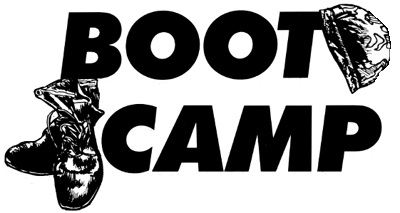by TOM HUDSON

With this issue, ANALOG begins a new column. Boot Camp will examine assembly language on the ATARI computer systems, while presenting useful subroutines to illustrate the techniques discussed in the column.

The Ground Rules
Before starting to learn assembly language, let's lay down the ground rules.
First, you should have a good reference guide to assembly-language operation codes. I suggest 6502 Assembly-Language Programming by Lance Leventhal (OSBORNE/McGraw-Hill). Of course, there are many such books, and the final choice of what book to use is up to you. Just be sure it covers the 6502 operation codes clearly and completely.
Second, since many program concepts will be shown in BASIC, you should have a working knowledge of BASIC. Assembly language requires a solid background in programming logic, and working in BASIC helps develop this skill. In addition, assembly-programming concepts can be grasped more easily if they are first shown in a language the reader is familiar with, such as BASIC. This should not be a problem for most readers, since BASIC is usually the first language learned by personal-computer owners. Therefore, from this point on, I will assume that all readers of this column are fluent in BASIC.
Third, you will need an assembler/editor package. All assembly-language listings in this column will be compatible with the ATARI Assembler/Editor cartridge and OSS's EASMD and MAC/65 assemblers. You can use other assemblers, but some code conversion may be necessary.
Fourth, you should be able to read flowcharts. Flowcharts are a good way to visualize a program's operation before actually writing any code.

Numbering Systems
Everybody is familiar with the decimal numbering system. We all use this numbering system in everyday mathematical calculations. The word "decimal" is derived from the Latin decem, or ten. Therefore, this numbering system is known as "Base 10", since there are ten digits, 0-9. Let's take a closer look at the decimal numbering system. Figure 1 shows how a six-digit Base 10 number can be broken down into individual digits. Each digit can range from 0-9 in value.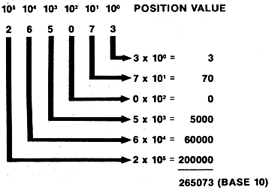Figure 1

Above each digit is that digit's position value. The position value is the amount each digit is multiplied by to get the actual value of the digit. You will notice that the position values are shown in powers of 10, since we are working in Base 10. The 1's position is shown as 10 to the 0 power. Any time a number is raised to the 0 power, the result is 1. Therefore, to get the value of the 3 in the last position of the number, we would calculate:
DIGIT x POSITION VALUE = VALUE

In this case the calculation would be:
3x1 =3
We would conclude that the last position in the number would have a value of 3.
The next position, containing the digit 7, has a position value of 10 to the first power, or 10. The calculation of this digit's value would be:
7x10=70
When we repeat this calculation for each digit in the number and add all the values, we will obtain the value of the number, 265,073 (Base 10).
Here's another concept that we may not think about, but is very interesting. What happens to the number if we shift all the digits to the left, as shown in Figure 2?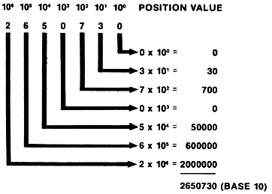Figure 2

By looking at the final results, you can see that the number has effectively been multiplied by 10, with a result of 2,650,730 (Base 10)!
Why did this happen? The answer is actually very simple. When each digit is shifted to the left, its position value is increased by a power of 10. The resulting number will be ten times larger than if it were not shifted. Try shifting the number to the right and see what happens.

What Do We Care About All This?
Now that we know exactly how our normal numbering system works, let's apply what we know to a different system, binary.
The word "binary" comes from the Latin bis, or "double". As you may know, digital computers work with two electrical states, on and off. This situation is perfectly suited for the binary numbering system, or Base 2.
The binary numbering system uses only two digits, 0 and 1, but the principle of the numbering system is the same as Base 10. Figure 3 shows a number in Base 2 and how it can be converted to Base 10.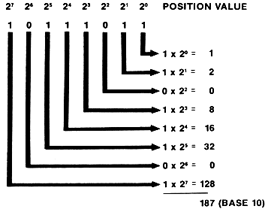Figure 3

Once Again, the number is shown with the position values above each digit in the number. In Base 2, you will notice that the position values are powers of 2. This means that, unlike the decimal progression of 1,10,100, etc. the binary system has a progression of 1, 2, 4, 8 and so on. As a result, the number 10111011 (Base 2) is 187 in Base 10. Figure 4 shows the binary equivalents of the numbers 0-19. Try using the method shown in Figure 3 to convert these numbers to the Base 10 equivalents shown.

 BASE 10 BASE 2 BASE 10 BASE 2 0 1 2 3 4 5 6 7 8 9 0 1 10 11 100 101 110 111 1000 1001 10 11 12 13 14 15 16 17 18 19 1010 1011 1100 1101 1110 1111 10000 10001 10011 10011

Figure 4

Remember how a Base 10 number multiplied by 10 when we shifted it left one digit? Let's look at how a binary number is affected by such a shift. Figure 5a shows the number 7 in binary before the shift and Figure 5b shows the number after the shift.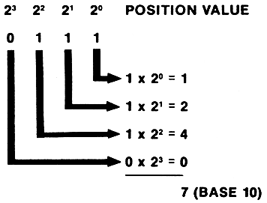Figure 5a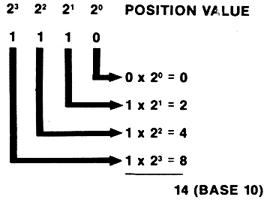Figure 5b

The number has been multiplied by 2! By examining this result and the above shift in Base 10, we can see that by shifting the digits in a number left to right, we multiply or divide the number by its base number. This concept will come in very handy in later installments of this column, so keep it in mind.

"Funny" Numbers
The mechanics of the binary numbering system are extremely important, but they can cause some problems.
Let's say you want to look at what is in Memory Location 33011, but don't want to give the number in decimal for some reason. The most logical choice, as far as your computer is concerned, is binary. Unfortunately for us humans, this number comes out as 1000000011110011, and is cumbersome, to say the least. We don't like to handle numbers like this-there are just too many chances to make a mistake.
Fear not! There is yet another numbering system that is compatible with both our friend the computer and our human limitation for handling large numbers. What is this system, you ask? It is called Base 16, or hexadecimal.
We have already noted that Base 10 uses ten digits, 0-9, and that Base 2 uses two digits, 0-1. Naturally, then, it follows that Base 16 uses sixteen digits.
But wait a minute! Since we humans normally use only the ten digits from the decimal system, we don't have enough for Base 16-we'll have to come up with six more. Rather than invent six new digit symbols, we'll use the letters A-F, which are already in existence. Figure 6 shows the 16 digits used in hexadecimal and their decimal equivalents.

 BASE 10 BASE 16 0 1 2 3 4 5 6 7 8 9 10 11 12 13 14 15 0 1 2 3 4 5 6 7 8 9 A B C D E F

Figure 6

Once again, the principle of the hexadecimal (hex) numbering system is the same as the other systems we have examined so far; the only difference is that the letters A-F must be thought of as the numbers 10-15. Figure 7 shows the conversion of the hex number \$F4BE (all hex numbers should be preceded by a "\$") to decimal.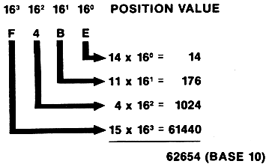Figure 7

So how does expressing numbers in hex help us avoid binary monstrosities? It's easy. The number 33011 (1000000011110011 in binary) is \$80F3 in hex. Obviously, this is a much easier number to remember than its binary equivalent.
Another interesting fact is that binary numbers are very easy to convert to hex. First the binary number must be divided into groups of four digits, from right to left. Then each group of four digits (ranging in value from 0-15) can easily be converted to the corresponding hex digit 0-F. Figure 8 illustrates this technique.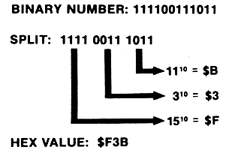Figure 8

Bytes and Bits
All readers who have owned their computers for more than a few days have at least heard of the terms "byte" and "bit". Usually this term pops up when the memory capacity of the computer is being discussed.
The byte is the unit most often used when referring to memory size. If your system has "16K" of memory, it has 16 x 1024 bytes, or 16384 bytes total. Each byte is made up of eight bits (short for binary digits). Each bit can be either off or on, corresponding to the 0 and 1 digits in the binary numbering system. With eight digits, this means that each byte can have 2 to the 8th power (or 256) combinations. This is why BASIC limits values in the POKE command to the range of 0-255.
In the process of learning assembly language, we will learn to manipulate the memory of your computer to do the things we want. Study these concepts carefully as they will be used in almost every assemblylanguage program you write.

How Assembly Works
In BASIC, a programmer can simply type in a program, type RUN, and the computer will begin executing the program immediately. If there is a problem, the programmer presses Break, finds the error and runs the program again. This makes programming very easy, and almost everyone is happy.
Yes, I said almost everyone.
Unfortunately for budding game programmers, a kind of brick wall soon appears on the happy road to the ultimate game. These programmers soon find that BASIC is far too slow to handle the complex graphics and game logic necessary for an arcade-style game. At the very least, assembly-language subroutines are necessary to speed things up.
Why is BASIC so slow? Inside,the computer is a device called a 6502 microprocessor. This little chip of silicon is what makes your computer work. It is capable of performing hundreds of thousands of operations per second, and does so every second your computer is powered on!
Sadly, all this computing power is lost as soon as a BASIC cartridge is inserted into your machine. You see, the microprocessor doesn't understand a single word of English, and the BASIC cartridge must act as an interpreter.
All of this interpreting takes time, and instead of doing the work you want, the poor microprocessor winds up spending most of its time translating BASIC into a language it can understand: binary. And this translation doesn't happen just once-it happens every time a BASIC command is executed! What a waste.
Assembly language, on the other hand, uses what is known as an assembler to perform this translation just once. The programmer writes a program in a special format. This is known as the source code. When ready to execute the program, the programmer processes it with an assembler, which translates the source code into object code, which is the actual binary machine-language. This code can be loaded at any time and executed as fast as the computer can go. It only has to be assembled once.
There are a few trade-offs involved when using assembly language, however.
First, the programmer must re-assemble a program each time a change is made. This can take quite a bit of time when a large program is involved. For this reason, it is a good idea to flowchart each program before writing any code. This helps reduce logic errors.
Second, the programmer must know where the program will be located in memory. Since the computer's operating system has certain needs, the programmer must be aware of what memory locations are available.
Third, errors can be hard to find. When a program is executing at hundreds of thousands of operations per second, an error cannot always be easily traced to a certain instruction. For this reason, a good debugging package is a must.
Fourth, all arithmetic must be handled explicitly by the programmer. Assembly language does not have square root, sine or cosine functions. It cannot multiply or even divide! Unless the programmer specifies otherwise, the addition and subtraction instructions can only produce numbers from -128 to 127. In the course of this column, we will examine the arithmetic functions that are possible in assembly language and how they are coded.
This may sound like a lot of limitations, but the 6502 processor allows the programmer to use the computer's built-in operating system directly, which BASIC has a hard time doing. And, of course, assembly language can be thousands of times faster than BASIC, allowing the programmer to write real-time simulations and arcade-style games.
Now that we've laid the groundwork for assembly-language programming, let's look at the 6502 itself.

Chip Off the Old Block
The 6502 processor chip has six registers that we are concerned with. These registers hold specific information and provide work areas for the programmer, and are shown in Figure 9.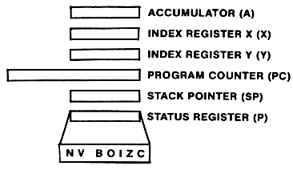Figure 9

The accumulator (A) is the most important register as far as the programmer is concerned. It is used for all arithmetic operations and most data manipulation. The accumulator is used more than any other register.
The index registers (X and Y) are used to hold memory indexes, counters, or offsets into tables. They can also be used as temporary storage areas.
The program counter (PC) is used by the 6502 to keep track of what instruction is being executed. This register is 16 bits long, enabling it to point to any byte in memory (up to 65535, or 64K). Since this register is maintained by the 6502, we will not be referencing it very often.
The stack pointer (SP) is used by the 6502 to keep track of a temporary storage region known as the stack. The stack holds subroutine return addresses and other temporary data. Since this registration is maintained by the 6502, we will not be referencing it very often, either.
The processor status register (P) is made up of seven individual "flags", or indicators, which inform the programmer of the 6502's current status.
The sign flat (N) is 0 when the result of an operation is positive, and 1 when the result is negative.
The overflow flag (V) is set to the exclusive-or of Bits 6 and 7 of the result of an arithmetic operation. The exclusive-or will result in a TRUE result if either bit being evaluated is TRUE, but not if both are TRUE. The overflow flag is rarely used, and is not important at this point.
The break flag (B) is set to 1 when a BRK instruction is executed. We will be using the instruction during program testing to stop program execution.
The decimal mode (D) flag is used to tell the processor to use either binary (0) or binary-coded decimal (1) arithmetic. This flag is important, and the programmer must be aware of its setting at all times.
The interrupt flat (I) enables or disables system-interrupts, depending on its setting.
The zero flag (Z) is set to 1 when any arithmetic or logical operation produces a zero result. A non-zero result sets the flag to 0. The carry flag (C) holds carries out of add, shift, and rotate instructions. It is also used as a borrow flag in subtraction operations. This is a very important flag, and will be discussed in detail later.
Next issue, we'll cover the different ways instructions can address memory, and start studying arithmetic operations, subroutines, and several other areas. Until then, study what has been covered here until you understand it thoroughly.